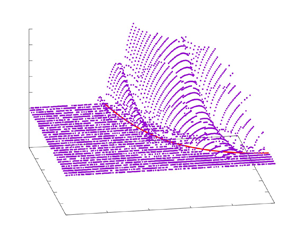Hostname: page-component-797576ffbb-xg4rj Total loading time: 0 Render date: 2023-12-01T14:10:41.141Z Has data issue: false Feature Flags: { "corePageComponentGetUserInfoFromSharedSession": true, "coreDisableEcommerce": false, "useRatesEcommerce": true } hasContentIssue false

## AbstractWe explore basic mechanisms for the instability of finite-amplitude interfacial gravity waves through a two-dimensional linear stability analysis of the periodic and irrotational plane motion of the interface between two unbounded homogeneous fluids of different density in the absence of background currents. The flow domains are conformally mapped into two half-planes, where the time-varying interface is always mapped onto the real axis. This unsteady conformal mapping technique with a suitable representation of the interface reduces the linear stability problem to a generalized eigenvalue problem, and allows us to accurately compute the growth rates of unstable disturbances superimposed on steady waves for a wide range of parameters. Numerical results show that the wave-induced Kelvin–Helmholtz (KH) instability due to the tangential velocity jump across the interface produced by the steady waves is the major instability mechanism. Any disturbances whose dominant wavenumbers are greater than a critical value grow exponentially. This critical wavenumber that depends on the steady wave steepness and the density ratio can be approximated by a local KH stability analysis, where the spatial variation of the wave-induced currents is neglected. It is shown, however, that the growth rates need to be found numerically with care and the successive collisions of eigenvalues result in the wave-induced KH instability. In addition, the present study extends the previous results for the small-wavenumber instability, such as modulational instability, of relatively small-amplitude steady waves to finite-amplitude ones.

## JFM classification

Type
JFM Papers
Information
Journal of Fluid Mechanics , 10 May 2022 , A13

## Access options

Get access to the full version of this content by using one of the access options below. (Log in options will check for institutional or personal access. Content may require purchase if you do not have access.)

## References

#### REFERENCES

Baker, G.R., Meiron, D.I. & Orszag, S.A. 1982 Generalized vortex methods for free-surface flow problems. J. Fluid Mech. 123, 477501.CrossRefGoogle Scholar
Benjamin, T.B. & Bridges, T.J. 1997 Reappraisal of the Kelvin–Helmholtz problem. Part 2. Interaction of the Kelvin–Helmholtz, superharmonic and Benjamin–Feir instabilities. J. Fluid Mech. 333, 327373.CrossRefGoogle Scholar
Choi, W. & Camassa, R. 1999 Exact evolution equations for surface waves. J. Engng Mech. ASCE 125, 756760.CrossRefGoogle Scholar
Dixon, A. 1990 A stability analysis for interfacial waves using Zakharov equation. J. Fluid Mech. 214, 185210.CrossRefGoogle Scholar
Dyachenko, A.L., Zakharov, V.E. & Kuznetsov, E.A. 1996 Analytical description of the free surface dynamics of an ideal fluid (canonical formalism and conformal mapping). Phys. Lett. A 221, 7379.CrossRefGoogle Scholar
Drazin, P.G. & Reid, W.H. 1981 Hydrodynamic Stability. Dover.Google Scholar
Grimshaw, R.H.J. & Pullin, D.I. 1985 Stability of finite-amplitude interfacial waves. Part 1. Modulational instability for small-amplitude waves. J. Fluid Mech. 160, 297315.CrossRefGoogle Scholar
Grue, J., Friis, A., Palm, E. & Rusås, P.O. 1997 A method for computing unsteady fully nonlinear interfacial waves. J. Fluid Mech. 351, 223252.CrossRefGoogle Scholar
Holyer, J.Y. 1979 Large amplitude progressive interfacial waves. J. Fluid Mech. 93, 433448.CrossRefGoogle Scholar
Lamb, H. 1945 Hydrodynamics, 6th edn. Dover.Google Scholar
Longuet-Higgins, M.S. & Tanaka, M. 1997 On the crest instabilities of steep surface waves. J. Fluid Mech. 336, 5168.CrossRefGoogle Scholar
MacKay, R.S. & Saffman, P.G. 1986 Stability of water waves. Proc. R. Soc. Lond. A 406, 115125.Google Scholar
McLean, J.W. 1982 Instabilities of finite-amplitude water waves. J. Fluid Mech. 114, 315330.CrossRefGoogle Scholar
Mei, C.C., Stiassnie, M. & Yue, D.K.P. 2005 Theory and Applications of Ocean Surface Waves, Part 2: Nonlinear Aspects. World Scientific.Google Scholar
Meiron, D.I. & Saffman, P.G. 1983 Overhanging interfacial gravity waves of large amplitude. J. Fluid Mech. 129, 213218.CrossRefGoogle Scholar
Murashige, S. & Choi, W. 2020 Stability analysis of deep-water waves on a linear shear current using unsteady conformal mapping. J. Fluid Mech. 885, A41.CrossRefGoogle Scholar
Ovshannikov, L. 1974 To the shallow water theory foundation. Arch. Mech. 26, 407422.Google Scholar
Părău, E. & Dias, F. 2001 Interfacial periodic waves of permanent form with free-surface boundary conditions. J. Fluid Mech. 437, 325336.CrossRefGoogle Scholar
Pullin, D.I. & Grimshaw, R.H.J. 1985 Stability of finite-amplitude interfacial waves. Part 2. Numerical results. J. Fluid Mech. 160, 317336.CrossRefGoogle Scholar
Saffman, P.G. & Yuen, H.C. 1982 Finite-amplitude interfacial waves in the presence of a current. J. Fluid Mech. 123, 459476.CrossRefGoogle Scholar
Tanaka, M. 1983 The stability of steep gravity waves. J. Phys. Soc. Japan 52, 30473055.CrossRefGoogle Scholar
Tanveer, S. 1991 Singularities in water waves and Rayleigh–Taylor instability. Proc. R. Soc. Lond. A 435, 137158.Google Scholar
Tiron, R. & Choi, W. 2012 Linear stability of finite-amplitude capillary waves on water of infinite depth. J. Fluid Mech. 696, 402422.CrossRefGoogle Scholar
Tsuji, Y. & Nagata, Y. 1973 Stokes’ expansion of internal deep water waves to the fifth order. J. Oceanogr. Soc. Japan 29, 6169.Google Scholar
Turner, R.E.L. & Vanden-Broeck, J.-M. 1986 The limiting configuration of interfacial gravity waves. Phys. Fluids 29, 372375.CrossRefGoogle Scholar
Vanden-Broeck, J.-M. 1980 Numerical calculation of gravity-capillary interfacial waves of finite amplitude. Phys. Fluids 23, 17231726.CrossRefGoogle Scholar
Yuen, H. 1984 Nonlinear dynamics of interfacial waves. Physica D 12, 7182.CrossRefGoogle Scholar
Zhou, C.P., Lee, J.H.W. & Cheung, Y.K. 1992 Instabilities and bifurcations of interfacial water waves. Phys. Fluids A 4, 14281438.CrossRefGoogle Scholar